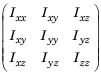Documentation

## Robot Dynamics

Robot dynamics is the relationship between the forces acting on a robot and the resulting motion of the robot. In Robotics System Toolbox™, manipulator dynamics information is contained within a `rigidBodyTree` object. This object describes a rigid body tree model that has multiple `rigidBody` objects connected through `rigidBodyJoint` objects. The `rigidBodyJoint`, `rigidBody`, and `rigidBodyTree` objects all contain information related to the robot kinematics and dynamics.

### Note

To use dynamics functions, you must set the `DataFormat` property to `'row'` or `'column'`. This setting takes inputs and gives outputs as row or column vectors for relevant robotics calculations, such as robot configurations or joint torques.

### Dynamics Properties

When working with robot dynamics, specify the information for individual bodies of your manipulator robot using properties on the `rigidBody` objects:

• `Mass` — Mass of the rigid body in kilograms.

• `CenterOfMass` — Center of mass position of the rigid body, specified as an `[x y z]` vector. The vector describes the location of the center of mass relative to the body frame in meters.

• `Inertia` — Inertia of rigid body, specified as an ```[Ixx Iyy Izz Iyz Ixz Ixy]``` vector relative to the body frame in kilogram square meters. The first three elements of the vector are the diagonal elements of the inertia tensor (moment of inertia). The last three elements are the off-diagonal elements of the inertia tensor (product of inertia). The inertia tensor is a positive definite matrix:For information related to your whole manipulator robot model, specify these `RigidBodyTree` object properties:

• `Gravity` — Gravitational acceleration experienced by the robot, specified as an `[x y z]` vector in meters per second squared. By default, there is no gravitational acceleration.

• `DataFormat` — The input and output data format for the kinematics and dynamics functions. Set this property to `'row'` or `'column'` to use dynamics functions. This setting takes inputs and gives outputs as row or column vectors for relevant robotics calculations, such as robot configurations or joint torques.

### Dynamics Functions

The following dynamics functions are available for robot manipulators. You can use these functions after specifying all the relevant dynamics properties on your `rigidBodyTree` robot model.